Transfer Function

# Transfer Function - Control Systems - Electrical Engineering (EE)

 Table of contentsConvolution TheoremTransfer Functions for Linear SystemsThe Concept of Pole & ZeroTransfer Function of Closed Loop SystemThe Transfer Function (TF) of a System is the ratio of the output to the input of a system, in the Laplace domain considering its initial conditions and equilibrium point to be zero.
If we have an input function of X(s), and an output function Y(s), we deﬁne the transfer function H(s).
H(s) = Y(s)/X(s)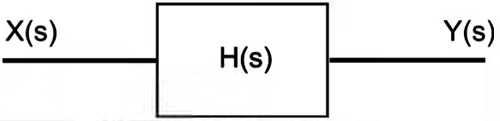### Impulse Response

Since we know that in the time domain, generally, we define the input to a system as x(t), and the output of the system as y(t). The relationship between the input and the output is represented as the impulse response, h(t).
We can use the following equation to deﬁne the impulse response:
h(t) = y(t) / x(t)

• The Impulse Function, denoted with δ(t) is a function deﬁned piece-wise as follows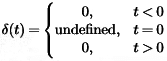• An examination of the impulse function shows that it is related to the unit-step function as follows
u(t) = ∫δ(t) or δ(t) = du(t) / dt
• The impulse response (IR) must always satisfy the following condition, or else it is not a true impulse function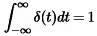Convolution in Time Domain

If we have the system input and the impulse response of the system, we can calculate the system output using the convolution operation as such as
y(t) = h(t) ∗ x(t).

Time-Invariant System Response

• If the system is time-invariant, then the general description of the system can be replaced by a convolution integral of the system's impulse response and the system input. We can call this the convolution description of a system.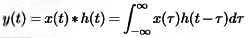## Convolution Theorem

• Convolution in the time domain turns into multiplication in the complex Laplace domain.
• Multiplication in the time domain turns into convolution in the complex Laplace domain.
L[f(t) ∗ g(t)] = F(s)G(s)
L[f(t)g(t)] = F(s) ∗ G(s)
Result: If the complex Laplace variable is s, then we generally denote the transfer function of a system as either G(s) or H(s). If the system input is X(s), and the system output is Y(s), then the transfer function can be deﬁned as such
H(s) = Y(s)/X(s)
• So if we know the input to a given system, and we have the transfer function of the system, we can solve for the system output by multiplying as
Y(s) = H(s)X(s)

Example: Impulse Response

• Since we know that the Laplace transform of the impulse function, δ(t) is L[δ(t)] = 1
• So, when we put this into the relationship between the input, output, and transfer function, we get
Y(s) = X(s)H(s)
or Y(s) = (1)H(s)
or Y(s) = H(s)
In other words, the "impulse response" is the o/p of the system when we input an impulse function.

Question for Transfer Function
Try yourself:
What is the transfer function of a system?

## Transfer Functions for Linear Systems

Consider a linear input/output system described by the controlled differential equation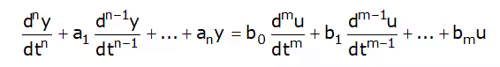where u is the input and y are the output.

• To obtain the transfer function of the system of last equation let us input be u(t) = est.
• Since the system is linear, there is an output of the system that is also an exponential function  ⇒ y(t) = Yoest
• Inserting the signals into last equation ,we ﬁnd
(sn + a1sn−1 +···+ an)yo.est = (bosm + b1sm−1···+ bm)e−st
• And the response of the system can be completely described as
a(s) = sn + a1sn−1 +···+ an &  b(s) = bosm + b1sm−1 + ···+ bm
y(t) = y0est = (b(s) / a(s))est
• So the transfer function of the given function is given by
G(s) = b(s) / a(s)

### Transfer Function Alternative Method

To investigate how a linear system responds to the exponential input u(t) = est we consider the state space system
dx/dt = Ax + Bu
y = Cx + Du
Let the input signal be u(t) = est and assume that s ≠ λi(A),  where i = 1,...,n, where λi(A) is the ith eigenvalue of A. then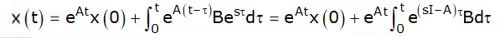Since s ≠ λ(A) the integral can be evaluated and we get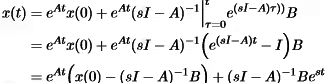& Output y(t) = Cx(t) + Du(t)
= CeAt{x(0) − (sI − A)−1B} + [D + C(sI − A)−1B]est
Note: One term of the output is proportional to the input u(t) = est. This term is called the pure exponential response.

• If the initial state is chosen as x(0) = (sI −A)−1B
• the output only consists of the only exponential response and both the state and the output are directly proportional to the input
x(t) = (sI − A)−1Best = (sI − A)−1Bu(t)
y(t) = [C(sI − A)−1B+D]est = [C(sI − A)−1B + D]u(t).
• The ratio of the output and the input
G(s) = C(sI − A)−1B + D is the transfer function of the system.
• For Homogeneous Equation i.e; D = 0 then
G(s) = C(sI − A)−1B  is the transfer function of the system.

## The Concept of Pole & Zero

• Poles and Zeros of a transfer function are the frequencies for which the value of the transfer function becomes inﬁnity or zero respectively.
• The transfer function has many useful explanations and the features of a transfer function are often associated with important system properties. Three of the most important features are the gain and the locations of the poles and zeros.
H(s) = N(s)/D(s)
• In the above equation, N(s) and D(s) are simple polynomials in s. Zeros are the roots of N(s) by setting N(s) = 0 and solving for s. Poles are the roots of D(s), by setting D(s) = 0 and solving for s.
• In general Transfer Function must not have more zeros than poles, we can state that the polynomial order of D(s) must be greater than or equal to the polynomial order of N(s).

Eﬀects of Poles and Zeros

• when s approaches a zero (Zeros of Transfer function), the numerator of the transfer function N(s)→0.
• When s approaches a pole (Poles of Transfer Function) the denominator of the transfer function D(s)→0 & the value of the transfer function approaches inﬁnity.
• An output value of inﬁnity should raise an alarm bell for people who are familiar with BIBO stability.
• Poles & Zeros of a transfer function are represented in S-Plane, In s-plane s = σ ± jω  where σ represent the attenuation on X-axis & ω  represents the angular velocity represented on the y-axis.
• In s-plane, the Poles are located by a cross (*) & the Zeros are located by dot (.)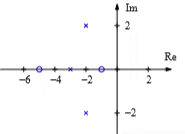• For a Stable System, All the Poles & Zeros of a Transfer Function must be lie in the left half of s-plane.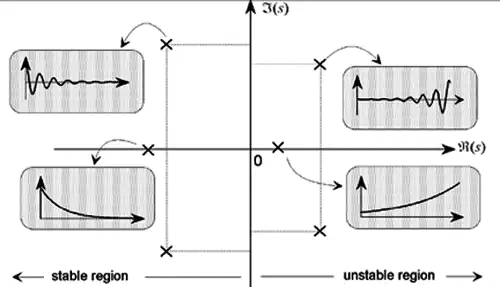Note: If the Poles or Zero lie on the imaginary axis then it must be simple (order only 1) then the system is said to be Marginally Stable, If the Order of multiplicity of Poles or Zeros on Imaginary Axis is more than 1 in that case system will become Unstable.

## Transfer Function of Closed Loop System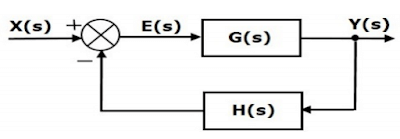Block diagram of Closed Loop SystemE(s) = Actuating or Error Signal

X(s) = Reference Input Signal.

G(s) = Forward Path Transfer Function.

Y(s) = Output Signal.

H(s) = Feedback Transfer Function.

B(s) = Feedback Signal.

So, the transfer function of the closed loop system is Y(s)/X(s).

From the block diagram,

Y(s) = G(s).E(s) .........1

B(s) = H(s).Y(s) .........2

E(s) = X(s) + B(s) .........3a    (For positive feedback)

= X(s) - B(s) ..........3b   (For negative feedback)

### For Negative Feedback (using eq.3b)

Put the value of E(s) from eq.3b in eq.1

Y(s) = G(s).[X(s)-B(s)]

Y(s) = G(s).X(s) - G(s).B(s) .........4

Put the value of B(s) from eq.2 in eq.4

Y(s) = G(s).X(s) - G(s).H(s).Y(s)

Y(s) + G(s).H(s).Y(s) = G(s).X(s)

Y(s){1 + G(s).H(s)} = G(s).X(s)

Y(s)/X(s) = G(s) / {1 +G(s).H(s)} .........5

### For Positive Feedback (using eq. 3a)

For positive feedback system we will use eq.3a and repeat the all the same steps and we will get the transfer function as:

Y(s)/X(s) = G(s) / {1 - G(s).H(s)} .........6

### For Unity Feedback

For unity feedback H(s) = 1, the eq.5 & eq.6 will become

Y(s)/X(s) = G(s)/{1 + G(s)} .......for negative unity feedback

Y(s)/X(s) = G(s)/{1 - G(s)} ........for positive unity feedback

The document Transfer Function | Control Systems - Electrical Engineering (EE) is a part of the Electrical Engineering (EE) Course Control Systems.
All you need of Electrical Engineering (EE) at this link: Electrical Engineering (EE)

54 docs|46 tests

## FAQs on Transfer Function - Control Systems - Electrical Engineering (EE)

 1. What is the Convolution Theorem in electrical engineering?Ans. The Convolution Theorem states that the Fourier Transform of the convolution of two signals is equal to the point-wise multiplication of their Fourier Transforms. In electrical engineering, this theorem is used to simplify the analysis of linear systems by allowing us to perform operations in the frequency domain instead of the time domain.
 2. How can the Transfer Function be used to analyze linear systems?Ans. The Transfer Function of a linear system is the ratio of the output to the input in the frequency domain. By analyzing the Transfer Function, we can determine the system's frequency response, stability, and other important characteristics. It allows us to predict the behavior of the system without the need for complex time-domain analysis.
 3. What is the significance of poles and zeros in a Transfer Function?Ans. Poles and zeros in a Transfer Function are important indicators of a system's behavior. Poles represent the frequencies at which the system response becomes infinite or unstable, while zeros represent the frequencies at which the response becomes zero or cancels out. By analyzing the locations of poles and zeros, we can determine the stability, frequency response, and other properties of the system.
 4. How does the Transfer Function of a closed-loop system differ from an open-loop system?Ans. The Transfer Function of a closed-loop system takes into account the feedback loop present in the system, whereas the Transfer Function of an open-loop system does not consider any feedback. In a closed-loop system, the output is fed back and compared to the desired input, allowing for better control and regulation. The closed-loop Transfer Function is typically more complex and involves the Transfer Functions of both the forward and feedback paths.
 5. How is the Transfer Function used in electrical engineering?Ans. The Transfer Function is widely used in electrical engineering for system analysis, design, and control. It allows engineers to analyze the behavior of linear systems in the frequency domain, assess stability and performance, design filters and equalizers, and determine the response to different inputs. The Transfer Function is a fundamental tool in various areas such as signal processing, communication systems, control systems, and circuit design.

## Control Systems

54 docs|46 testsExplore Courses for Electrical Engineering (EE) examSignup to see your scores go up within 7 days! Learn & Practice with 1000+ FREE Notes, Videos & Tests.
10M+ students study on EduRev
Track your progress, build streaks, highlight & save important lessons and more!
Related Searches

,

,

,

,

,

,

,

,

,

,

,

,

,

,

,

,

,

,

,

,

,

;